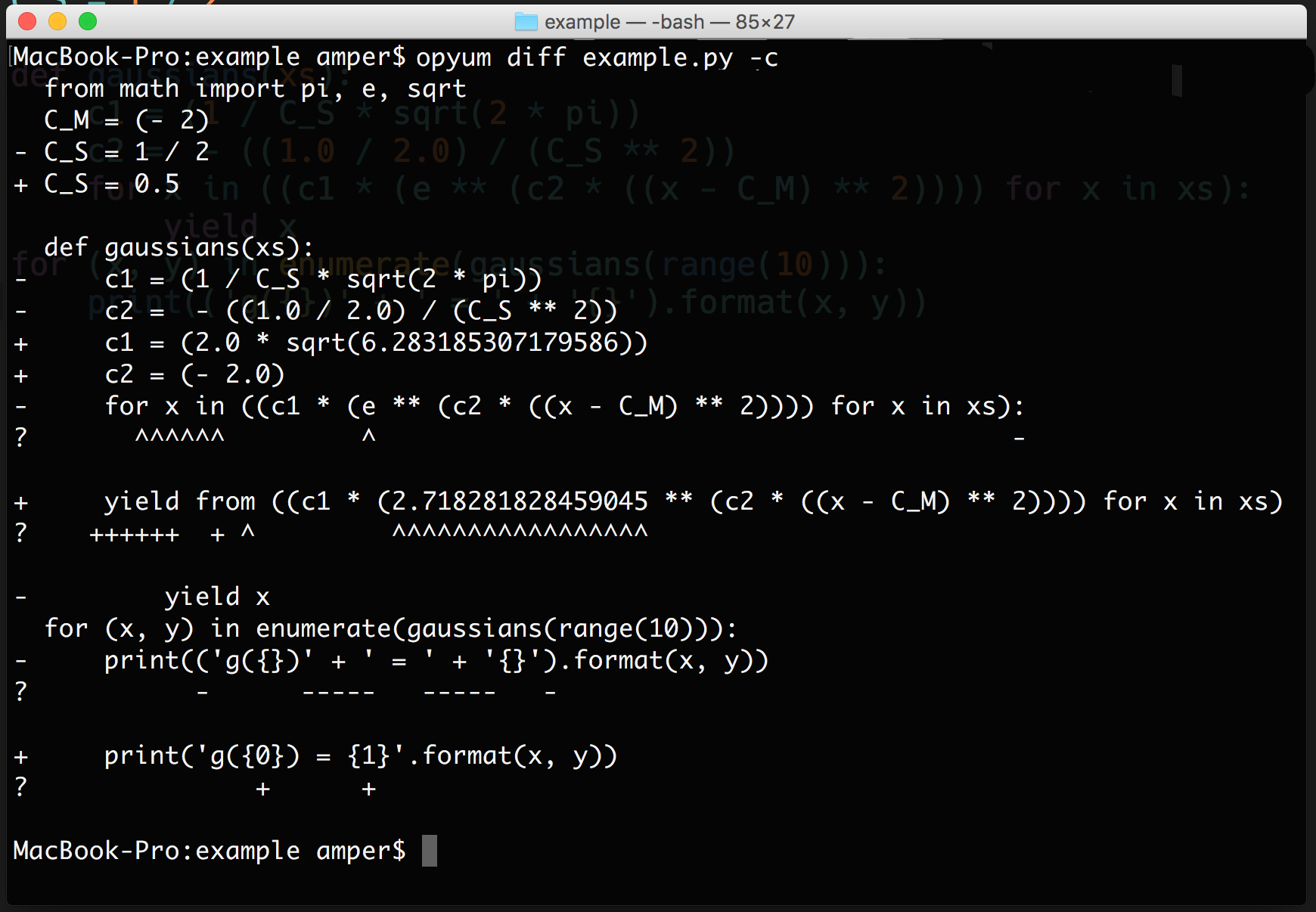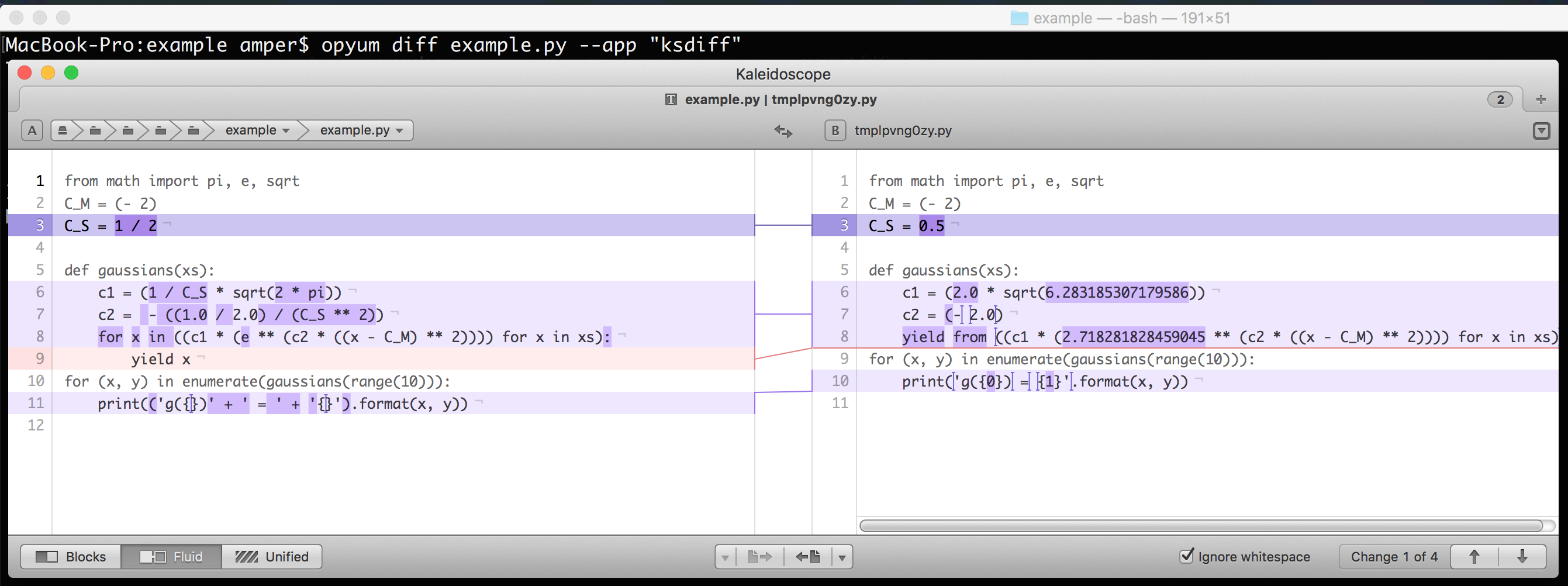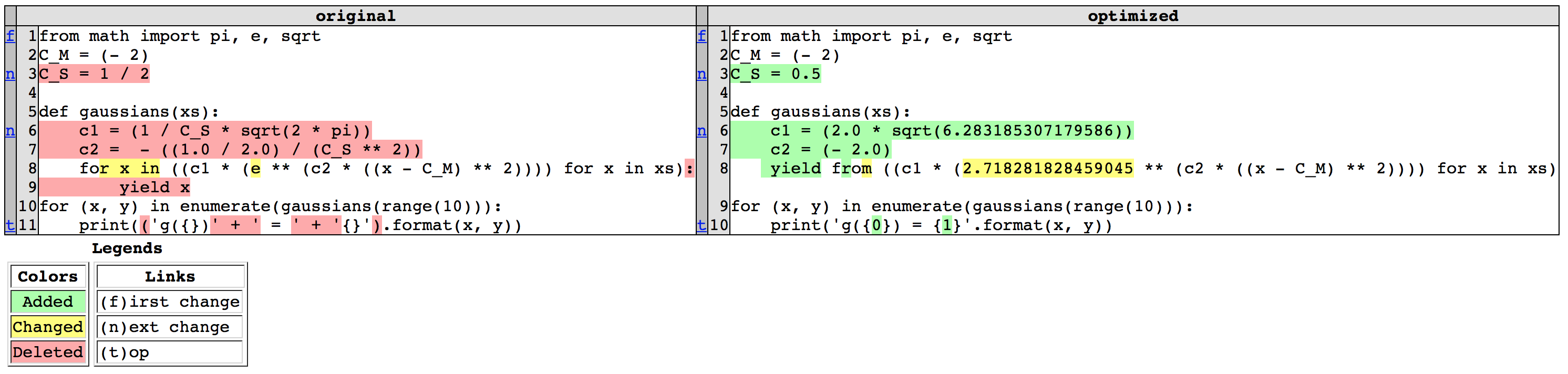# Amper/opyum

Optimizing Python applications without mutilation code
PythonFetching latest commit…
Cannot retrieve the latest commit at this time.
Type Name Latest commit message Commit time
Failed to load latest commit information.bindocsexampleopyumtests.coveragerc.gitignoreAUTHORSCHANGELOGINSTALLLICENSEMANIFEST.inREADME.mdREQUIREMENTS.txtsetup.pytox.ini

# opyum

## Description

Optimizing Python applications without mutilation code.

Use the automatic modification of AST for code optimization, which is transparent to the user and requires the addition of only a few lines.

## Usage

Decorator:

```from opyum import optimize

@optimize
def function_for_optimize():
...```

Import-hook:

```import opyum
opyum.activate()

# other imports```

"With" syntax:

```import opyum

with opyum.activate:
# other imports```

Command-line mode:

Show optimized source:

``````\$ opyum show myfile.py
``````

Diff between original source and optimized source:

``````\$ opyum diff myfile.py
``````

Console diff (with "-c" or "--console" option):Custom app diff (with "--app" option):By default, html diff (without options):## List of optimizations

####Constant folding

Before:

```x = 7 * 24 * 60 * 60
y = [i ** 2 for i in range(10) if i % 2 == 0]
z = sum(range(1000))```

After:

```x = 604800
y = [0, 4, 16, 36, 64]
z = 499500```

####"'Power' to 'multiplication'" optimization

Before:

```x1 = a ** (-2)
x2 = a ** (-1)
x3 = a ** ( 0)
x4 = a ** ( 1)
x5 = a ** ( 2)```

After:

```x1 = 1 / (a * a)
x2 = 1 / a
x3 = 1
x4 = a
x5 = a * a```

####"'Yield' to 'yield from'" optimization

Before:

```for x in some_expression:
yield x```

After

`yield from some_expression`

####Builtin constant propagation

Before:

```from math import pi
def circumference(r):
return 2 * pi * r```

After:

```from math import pi
def circumference(r):
return 2 * 3.141592653589793 * r```

####Custom constant propagation

Before:

```C_PI = 3.141592653589793

def circumference(r):
return 2 * C_PI * r```

After:

```C_PI = 3.141592653589793

def circumference(r):
return 2 * 3.141592653589793 * r```

Before:

```def do_something():
return 1
print('returning 1')

if condition1:
pass
elif condition2:
do_something()
else:
pass```

After:

```def do_something():
return 1

if not condition1 and condition2:
do_something()```

## Installation

Installation is simple with pip:

``````\$ pip install opyum
``````

or with setuptools:

``````\$ easy_install opyum
``````